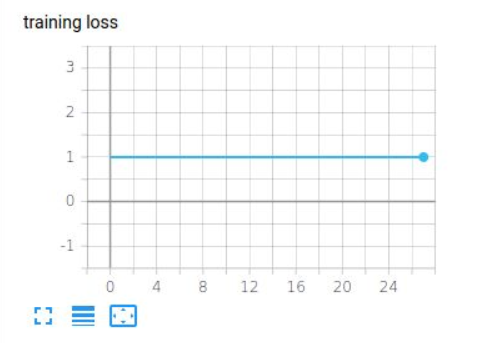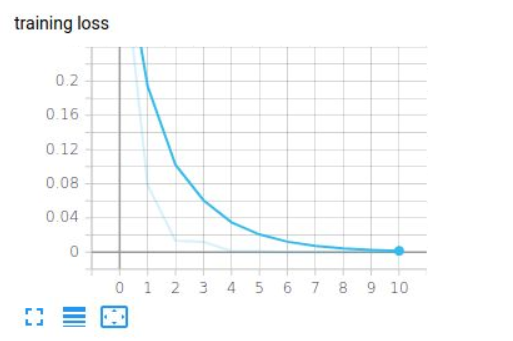# Including convolutional layers causes my network not to train

Hi all,

I’m trying to create a message-passing neural network to perform regression over graphs, and am implementing a model I found in a paper working in a similar domain. One section of this model, after message-passing has occurred, involves upsampling the number of features each node contains by two 1x1 convolutions (conv1, conv2 in my code).

I’ve been facing an error; however, where if I include these convolutions my network fails to train at all. All predicted values are 0 (even in a dataset where all targets are 1). When I remove the convolutional layers and all of the associated squeezing/unsqueezing code, my network trains fine and can overfit on a tiny dataset, but when they are included the loss doesn’t increase or decrease at all.

I’m at a loss as to what’s going wrong - does anyone know what might be causing this?

``````from torch_geometric.nn import global_max_pool as gmp

embed_dim = 128

# TODO: Apply dropout

class BuildingBlock(torch.nn.Module):
def __init__(self, in_channels, out_channels, dim=0):
super(BuildingBlock, self).__init__()
self.lin1 = Linear(in_channels, 256, dim)
self.hidden = Linear(256, 128)
self.lin2 = Linear(128, 128)

def forward(self, x):
x = F.relu(self.lin1(x))
x = F.relu(self.hidden(x))
x = F.dropout(x, 0.5)
x = F.relu(self.lin2(x))

return x

class PaliwalNet(torch.nn.Module):
def __init__(self, t):
super(PaliwalNet, self).__init__()

self.embedding = Embedding(num_embeddings=len(distinct_features)+1, embedding_dim=embed_dim)

self.MLP_V = BuildingBlock(embed_dim, 128)
self.MLP_E = BuildingBlock(1, 128)

self.message_passing_steps = nn.ModuleList()
for i in range(t):
self.message_passing_steps.append(PaliwalMP(embed_dim, embed_dim))

self.conv1 = nn.Conv1d(128, 512, (1,1))
self.conv2 = nn.Conv1d(512, 1024, (1,1))

# FCNN for final prediction
self.lin1 = Linear(1024, 512)
self.lin2 = Linear(512, 512)
self.lin3 = Linear(512, 256)
self.lin4 = Linear(256, 256)
self.lin5 = Linear(256, 128)
self.lin6 = Linear(128, 1)

def forward(self, data):
x, edge_index, edge_attr, batch = data.x, data.edge_index, data.edge_attr, data.batch
edge_index_u, edge_index_d = torch.split(edge_index, int(edge_index.shape/2), dim=1)
edge_attr_u, edge_attr_d = torch.split(edge_attr, int(edge_attr.shape/2))

# Generate learnable embeddings for node features
x = x.squeeze(-1)
x = self.embedding(x)

# Embed node and edge features into high dimensional space
x = self.MLP_V(x)
edge_attr_u = self.MLP_E(edge_attr_u.float())
edge_attr_d = self.MLP_E(edge_attr_d.float())

for message_passing_step in self.message_passing_steps:
x = message_passing_step(x, edge_index_u, edge_index_d, edge_attr_u, edge_attr_d)

x = x.unsqueeze(-1).unsqueeze(-1)
x = self.conv1(x)
x = self.conv2(x)

# Final prediction network
x = x.squeeze(-1).squeeze(-1)
g = gmp(x, batch)

g = F.relu(self.lin1(g))
g = F.relu(self.lin2(g))
g = F.relu(self.lin3(g))
g = F.relu(self.lin4(g))
g = F.relu(self.lin5(g))
g = F.relu(self.lin6(g))

return g
``````

Try to include batchnorm layer and activation layers in-between ur conv layers

Have just tried this, but no success sadly. Loss doesn’t change at all over training:I’m honestly not seeing what’s wrong with the code even tho I haven’t ran it for errors

Scan I see ur training loop?

Wait does ur dataset have negative values as targets?

The dataset only has 0’s and 1’s as targets. Having just reread the page for Conv1d, I suspect there might be an issue with the shape of my data being fed into the conv layer. Currently it’s in the form [n_nodes, 128] where n_nodes changes based on the input graph. It looks like the Conv1d expects [batch size, number of channels, sequence length], which I think in my case might be [1, 128, n_nodes]. Does that sound right?

Yah this is true
Ah alright! I’ll try it out and let you know whether it worksIt’s working now - thanks so much for the help mate. I’ll need to go and figure out what it was trying to do before that was messing it up!Nice!Well I wasn’t really of much help but well… Ur welcome